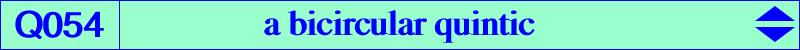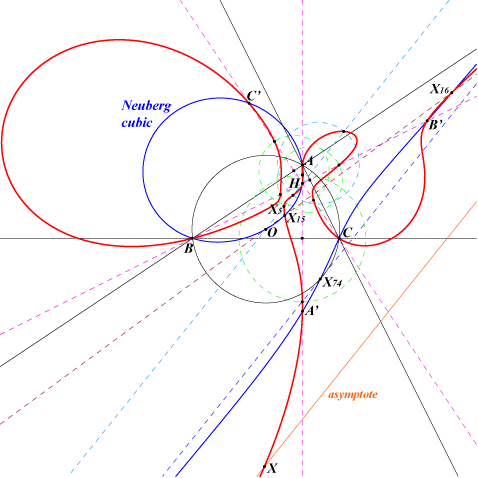too complicated to be written here. Click on the link to download a text file.X(4), X(5), X(15), X(16), X(30), X(5000), X(5001) A', B', C' reflections of A, B, C in the sidelines of ABC foci of the Steiner inellipseThe perpendiculars at B, C to the line BC meet the perpendicular bisectors of PB, PC at B1, C1. Let A' = BC1 /\ CB1 and define B', C' similarly. The triangles ABC and A'B'C' are perspective if and only if P lies on Q054. Q054 is a bicircular quintic with one real asymptote parallel to the Euler line, the homothetic of that of the Neuberg cubic under h(O,2). The last two points on the Euler line are X(5000), X(5001). They lie on the circle centered at X(468) orthogonal to the circumcircle. The last two points on the altitude AH are symmetric with respect to the line BC. Q037 and Q054 are both bicircular quintics which generate a pencil of quintics of the same type. One is decomposed into K018 and the line at infinity counted twice. See Table 55.